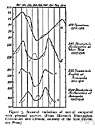# random variable

Also found in: Thesaurus, Medical, Financial, Acronyms, Encyclopedia, Wikipedia.

## random variable

n.
A variable whose values are random but whose statistical distribution is known.

## random variable

n
(Statistics) statistics a quantity that may take any of a range of values, either continuous or discrete, which cannot be predicted with certainty but only described probabilistically. Abbreviation: rv
Collins English Dictionary – Complete and Unabridged, 12th Edition 2014 © HarperCollins Publishers 1991, 1994, 1998, 2000, 2003, 2006, 2007, 2009, 2011, 2014

## ran′dom var′iable

n.
a statistical quantity that can take any of the values of a specified set in accordance with an associated probability distribution.
Also called variate.
[1935–40]
ThesaurusAntonymsRelated WordsSynonymsLegend:
 Noun 1random variable - a variable quantity that is random  variable quantity, variable - a quantity that can assume any of a set of values
Based on WordNet 3.0, Farlex clipart collection. © 2003-2012 Princeton University, Farlex Inc.
References in periodicals archive ?
This technique represents the random response of a model in terms of a linear combination of orthonormal polynomial basis involving the input random variables. Intrusive [3-5] or non-intrusive [2, 6, 7] approaches exist for the computation of the basis coefficients, thus reproducing an analytic representation called metamodel (or surrogate model) of the system response.
The vth moment of a random variable X, whose PDF f (X) is given, can be defined as
(3) The r-th moment of the random variable X is given by
Let [l.sub.i] and [[mu].sub.i] be the essential infimum and essential supremum of a random variable [X.sub.i], X = ([X.sub.1],..., [X.sub.n]) is a fixed random vector in [mathematical expression not reproducible], then X is mutually exclusive.
For h [member of] N and i between 0 and n, the moment [m.sub.ih] of order h of the random variable [[xi].sub.i] is defined by
Let X : [OMEGA] [right arrow] R be a random variable with finite expected value and let H [subset or equal to] S be a [sigma]-algebra.
If X(S) [??] R is at most countable in R, then X is a discrete random variable, otherwise, it is a continuous random variable.
However, this definition has never been used as the criterion for the assumption of a random variable in a macroeconometric model.
And G (u, [delta]) can be transformed to G(v) = 1 - [square root of ((2/(n + m)))] [v.sub.1][v.sub.2] in polar space, where the PDF of the random variable [v.sub.1] is (18), and the PDF of [v.sub.2] is (20).
The present goal is to derive the CDF and the PDF of the sum T = [[summation].sup.n.sub.i=1] [X.sub.i], where [X.sub.i] are independent identically distributed Uniform (0, 1) random variables for i = 1, 2, ..., n.

Site: Follow: Share:
Open / Close